Mobile QR Code1. (Department of Electrical Engineering, Pusan National University, Busan 46241, Korea)

SiC MOSFETs, short-circuit protection, short-circuit detection, soft termination circuit, soft turn off

## I. INTRODUCTION

Silicon carbide (SiC) metal-oxide-semiconductor field effect transistors (MOSFETs) can replace silicon insulated-gate bipolar transistors (IGBTs), which are widely utilized in electric vehicles (EVs) and the photovoltaic (PV) industry [1,2]. Such MOSFETs can operate with low losses, high-frequency, and have decent thermal characteristics [3-5]. Minimized low-weight power systems with the aforementioned characteristics can be fabricated using SiC MOSFETs . SiC MOSFETs have some differences compared with Si devices. For instance, SiC MOSFETs have low transconductance; therefore, they require a high driving voltage to reduce the on-state resistance. A negative voltage V$_{\mathrm{EE}}$ is used because of the low threshold voltage in the off state . Additionally, the overcurrent of an SiC MOSFET is faster and larger than that of Si IGBTs because of the high current density per unit area in the short-circuit (SC) states. The SC state refers to a case in which the high DC link voltage of the power system is directly connected to the ground of the system without passing through the load inductor. In other words, the high DC link voltage only passes through the power transistor during a SC state. A SC state can be caused by the destruction of the load, short-circuit patterns from external shock, fault gate control signal, and noise . The short circuit withstand time (SCWT) of SiC MOSFETs is approximately 3 ${\mathrm{\mu}}$s, which is significantly shorter than that of Si IGBTs . Therefore, SiC MOSFETs require a unique gate driver with a protection circuit for detecting faults to protect switching devices and circuits from a SC overcurrent.

The SC protection circuit is composed of SC detection and soft termination sections, as shown in Fig. 1. The role of an SC detection circuit is to locate faults as soon as possible within the SCWT to prevent the SC current from damaging the SiC MOSFET. Accordingly, a soft termination circuit can safely turn a device off and reduce damage. Importantly, short circuit detection circuits (SCDCs) should not affect the normal operation, switching, and conduction characteristics of the system, as well as area that of the printed circuit board (PCB). Moreover, SCDCs should be able to quickly locate an SC state.

In addition, they must operate stably without error signals and independent of switching noise in power systems. Various methods have been developed to detect the SC state.

Changes in the voltage and current waveforms between the normal and SC states can be used as an indicator to detect the SC state. An SCDC can detect faults by monitoring the gate-source voltage (V$_{\mathrm{GS}}$) [10,11], drain-source voltage (V$_{\mathrm{DS}}$) [12-15], and drain-source current (I$_{\mathrm{DS}}$) [16-19] of a SiC MOSFET.

The soft termination circuit (STC) is utilized to safely overcome the SC state. If the SiC MOSFET is abruptly turned off in the overcurrent state caused by the SC, a large dI$_{\mathrm{DS}}$/dt occurs and induces a V$_{\mathrm{DS}}$ spike that exceeds the breakdown voltage and has the potential to destroy a device . There are two types of STCs: soft-slope turn-off [21-23] and two-level turn-off [20,24]. A soft-slope turn-off circuit decreases the V$_{\mathrm{GS}}$ with a gentle slope when an SC is detected. The reduction of V$_{\mathrm{GS}}$ suppresses the rapid variation of I$_{\mathrm{DS}}$, which reduces the V$_{\mathrm{DS}}$ overshoot effect by preventing a high dI$_{\mathrm{DS}}$/dt. The two-level turn-off method reduces V$_{\mathrm{GS}}$ to V$_{\mathrm{GS}}$/2 to decrease the slope of dI$_{\mathrm{DS}}$/dt in fault situations.

##### Fig. 1. Short circuit protection circuit (SCPC).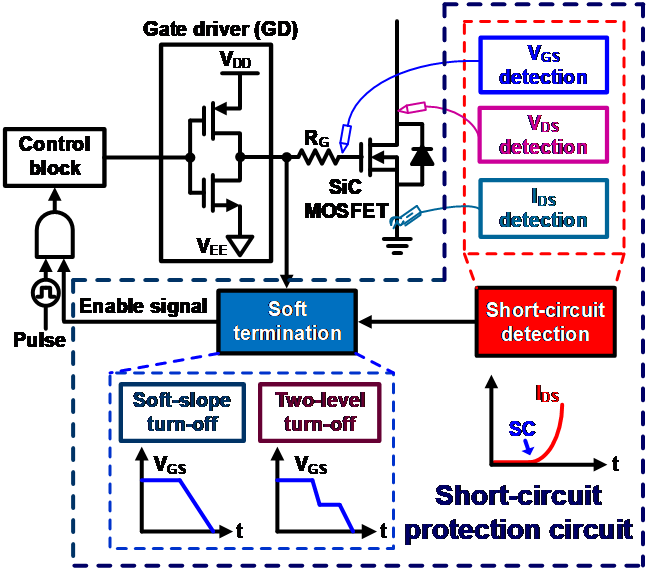This paper describes the principle, merits, and demerits of SCDCs and STCs. Moreover, considerations for designing an SCPC for a SiC MOSFET are introduced.

## II. SHORT CIRCUIT PROTECTION CIRCUITS

This section presents the causes of the SC states. Moreover, the principles of V$_{\mathrm{GS}}$, V$_{\mathrm{DS}}$, and I$_{\mathrm{DS}}$ waveforms are discussed. SC states are divided into hard-switching faults (HSF) and faults under loads (FUL).

If a SC state has already occurred before the switch is turned on, the SC state is called HSF [25,26]. The test bench circuit used for the SC test is displayed in Fig. 2. C$_{\mathrm{DC.link}}$ and L$_{\mathrm{load}}$ refer to the DC-link capacitor and inductor load in the power system, respectively. M$_{\mathrm{SC}}$ refers to a switch that passes the load to deliberately cause an SC state, and M$_{\mathrm{SiC}}$ refers to a SiC MOSFET, which is an actual switching device. Fig. 2 illustrates a test bench for the HSF state, where M$_{\mathrm{SiC}}$ is turned on when M$_{\mathrm{SC}}$ is on (during an SC). Additionally, the waveforms of V$_{\mathrm{GS.HSF}}$, V$_{\mathrm{DS.HSF}}$, I$_{\mathrm{DS.HSF}}$ in the HSF state are illustrated in Fig. 3, respectively . The gate-source voltage, drain-source current, and drain-source voltage in the normal state are denoted as V$_{\mathrm{GS.nor}}$, I$_{\mathrm{DS.nor}}$, V$_{\mathrm{DS.nor}}$, respectively. Because the V$_{\mathrm{DC.link}}$ is completely applied to the M$_{\mathrm{SiC}}$ while the load is ignored, even if V$_{\mathrm{GS}}$ increases and I$_{\mathrm{DS}}$ flows sufficiently, V$_{\mathrm{DS}}$ does not drop and maintains itself similar to V$_{\mathrm{DS.HSF}}$. Therefore, the Miller plateau region of V$_{\mathrm{GS}}$ does not appear, and the gate-source voltage rises, similar to the V$_{\mathrm{GS.HSF}}$ in the HSF . Once the SiC MOSFET is fully turned on, V$_{\mathrm{DS.HSF}}$ is maintained, and I$_{\mathrm{DS.HSF}}$ continues to increase without saturation. The SCDC should be able to detect faults quickly and must distinguish between a normal state and an SC state during the turn-on transition to protect the device and circuit in case of HSFs.

Fig. 4 displays the testbench for the FUL test. While the M$_{\mathrm{SiC}}$ operates normally, M$_{\mathrm{SC}}$ is turned on, resulting in the occurrence of the FUL state . In a normal state, I$_{\mathrm{DS.nor}}$ flows through L$_{\mathrm{load}}$. However, when FUL occurs (namely, when M$_{\mathrm{SC}}$ turns on), current flows similar to I$_{\mathrm{DS.FUL}}$. Fig. 5 depicts the gate-source voltage V$_{\mathrm{GS.FUL}}$, drain-source current I$_{\mathrm{DS.FUL}}$, and drain-source voltage V$_{\mathrm{DS.FUL}}$ in FUL, respectively. As shown in Fig. 5, M$_{\mathrm{SiC}}$ initially operates normally during t$_{\mathrm{normal}}$, and V$_{\mathrm{DS.FUL}}$ rises intentionally to turn on M$_{\mathrm{SC}}$ to start the fault, and ignores L$_{\mathrm{load}}$. At the time, some of the I$_{\mathrm{DS.FUL}}$ flows through the parasitic capacitance C$_{\mathrm{GD}}$ at the gate-drain of M$_{\mathrm{SiC}}$, and an overshoot voltage occurs at V$_{\mathrm{GS.FUL}}$. To detect the FUL, the SCDC must be able to detect the SC during conduction.

##### Fig. 2. Testbench of a hard switching fault (HSF).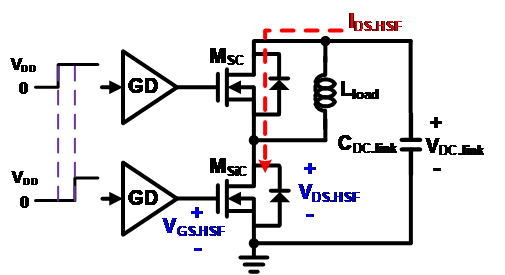##### Fig. 3. Waveforms of V$_{\mathrm{GS}}$, V$_{\mathrm{DS}}$, and I$_{\mathrm{DS}}$ in the normal and HSF states.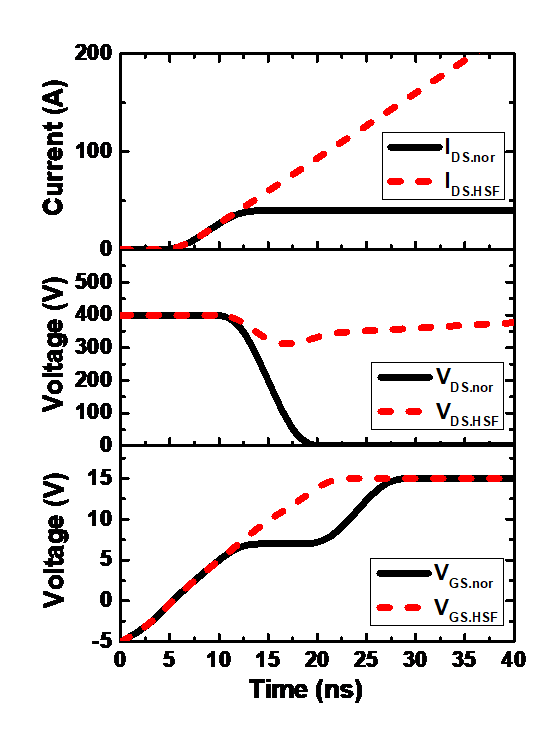##### Fig. 4. Testbench of fault under load (FUL).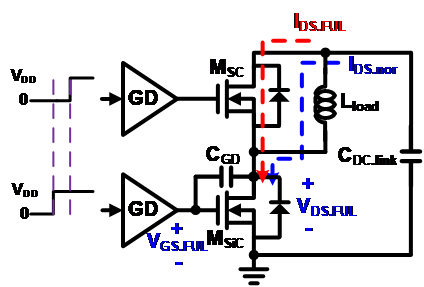##### Fig. 5. Waveforms of V$_{\mathrm{GS}}$, V$_{\mathrm{DS}}$, and I$_{\mathrm{DS}}$ in normal and FUL state.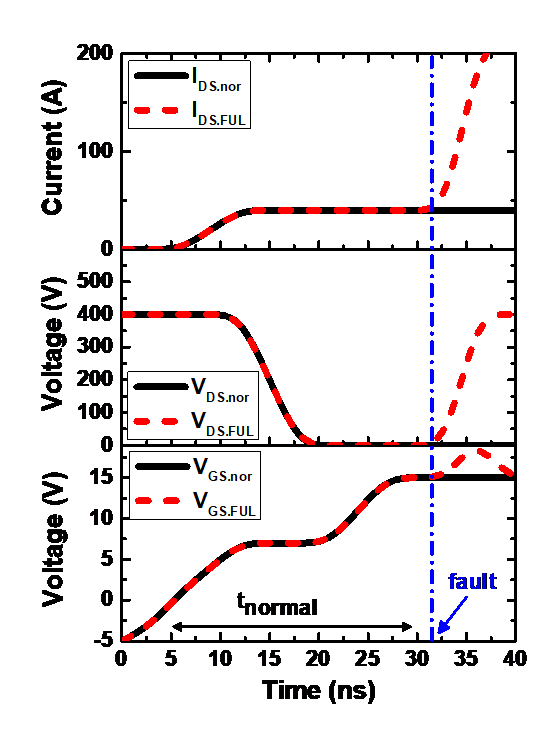### 1. Short Circuit Detection Circuits

The shapes of the voltage or current waveforms, which are different from those in the normal state, are used to detect the SC state. If the SC occurs because of an external shock or a fault in the gate signal, changes in V$_{\mathrm{GS}}$, V$_{\mathrm{DS}}$, and I$_{\mathrm{DS}}$ appear, as shown in Fig. 3 and 5. Numerous SCDCs have been studied using the shape change in each waveform [12,28].

#### A. Gate-source Voltage Detection Method

Fig. 6 shows a circuit that can detect an SC condition by monitoring V$_{\mathrm{GS}}$ [10,11]. The circuit determines the SC depending on whether a Miller plateau exists in turning on the transition for HSF. The FUL can be sensed when V$_{\mathrm{GS}}$ overshoots beyond the gate-driving voltage. In the HSF state, because V$_{\mathrm{DS.HSF}}$ does not decrease, like the waveform in Fig. 3, the Miller plateau of V$_{\mathrm{GS.HSF}}$ is not generated and immediately rises to the driving voltage of the gate driver. Therefore, the SC is determined when the value of V$_{\mathrm{GS}}$ exceeds the reference voltage V$_{\mathrm{th1}}$ of the comparator in the Miller plateau section. When V$_{\mathrm{DS.FUL}}$ increases because of the FUL state, an inrush current flows through I$_{\mathrm{DS.FUL}}$ through the C$_{\mathrm{GD}}$ of the SiC MOSFET, causing an overshoot of the voltage in the gate node. The comparator monitors V$_{\mathrm{GS.FUL}}$ by comparing it with V$_{\mathrm{th2}}$ to determine the SC state.

The V$_{\mathrm{GS}}$ detection method does not directly sense the main power path; hence, it does not affect normal operation. However, it is difficult to monitor the Miller plateau region during high speed operation. Additionally, high source and sink currents are required to reduce switching losses and these can occur noise at the gate node. To alleviate this problem, a filter, such as an integrator, can be employed to detect V$_{\mathrm{GS}}$.

##### Fig. 6. Conventional V$_{\mathrm{GS}}$ detection circuit for sensing the SC state.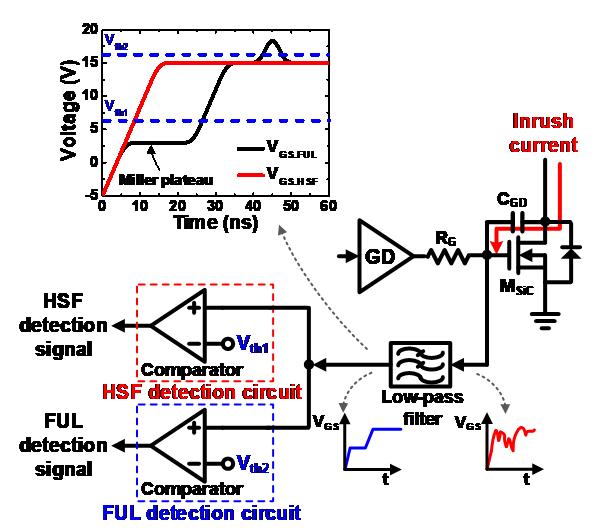#### B. Drain-source Voltage Detection Method

Desaturation detection is a representative method of the V$_{\mathrm{DS}}$ detection method, which is implemented as shown in Fig. 7 [12-15]. The desaturation detection method has been used in Si IGBT power systems for long, and it is now extensively utilized as an SCDC for SiC MOSFETs. As depicted in Fig. 7, the desaturation detection circuit comprises a high-voltage fast diode D$_{\mathrm{DESAT}}$, current source I$_{\mathrm{sense}}$, blanking capacitor C$_{\mathrm{blank}}$, and comparator.

The operating principle is as follows. When the M$_{\mathrm{SiC}}$ turns on, I$_{\mathrm{sense}}$ starts to flow simultaneously. In a normal state, V$_{\mathrm{DS}}$ is approximately 0 V and R$_{\mathrm{on}}$ (resistance of M$_{\mathrm{SiC}}$) is very small; therefore, I$_{\mathrm{sense}}$ flows to the ground via D$_{\mathrm{DESAT}}$ and M$_{\mathrm{SiC}}$. Thus, C$_{\mathrm{blank}}$ is not charged, the sense voltage V$_{\mathrm{DESAT}}$ is maintained at a low value, and the output of the comparator is low. In the SC state, as depicted in Fig. 7, V$_{\mathrm{DS.HSF}}$ is maintained at a high voltage and D$_{\mathrm{DESAT}}$ is turned off. Simultaneously, I$_{\mathrm{sense}}$ starts charging C$_{\mathrm{blank}}$, and when V$_{\mathrm{DESAT}}$ exceeds V$_{\mathrm{REF}}$, the output of the comparator becomes high, sending a SC protection signal (SCPS) to the controller.

The desaturation detection method to locate SCs is highly reliable because it has been previously used for protecting Si IGBTs from a SC state. Additionally, the desaturation detection method can perform detection at high speeds by adjusting the value of C$_{\mathrm{blank}}$. However, external devices such as D$_{\mathrm{DESAT}}$, R$_{1}$, and C$_{\mathrm{blank}}$ are required to detect an SC; therefore, the total area of the gate driver increases.

##### Fig. 7. Desaturation detection method circuit, a V$_{\mathrm{DS}}$ detection circuit for sensing the SC state.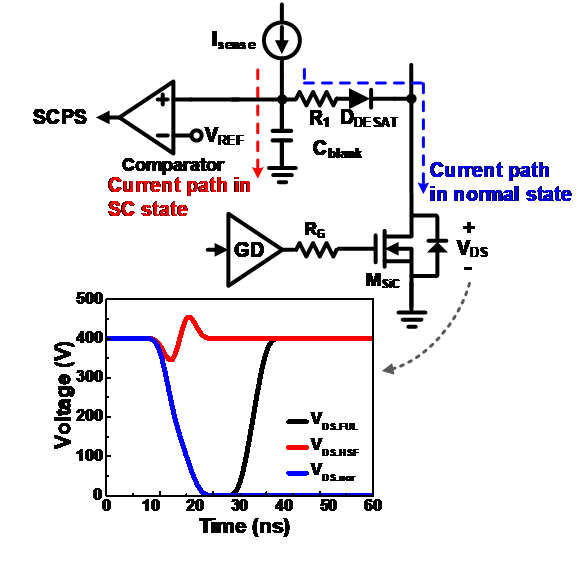#### C. Drain-source Current Detection Method

While the two aforementioned detection methods indirectly determine the SC state using the waveform changes of V$_{\mathrm{GS}}$ or V$_{\mathrm{DS}}$, the drain-source current I$_{\mathrm{DS}}$ detection method directly measures the magnitude of the SC current I$_{\mathrm{DS.SC}}$. The drain-source current detection method is suitable as an SCDC for SiC MOSFETs as it can determine the SC state swiftly and accurately. Therefore, numerous methods for detecting I$_{\mathrm{DS.SC}}$ have been proposed [16-19]. In the past, as shown in Fig. 8, the magnitude of the SC current was monitored by detecting the voltage V$_{\mathrm{shunt}}$ (= I$_{\mathrm{DS.SC}}$ ${\times}$ R$_{\mathrm{shunt}}$) induced by I$_{\mathrm{DS.SC}}$ flowing through the shunt resistor. However, the power loss caused by the resistor placed in the main current path is a considerable drawback of the method.

Another method uses SenseFET, as depicted in Fig. 9 . The SenseFET monitors the current flowing through the auxiliary transistor M$_{\mathrm{AUX}}$, which is several thousand times smaller in magnitude than that of the main transistor M$_{\mathrm{SiC}}$. Power loss can be reduced, and the current can be safely detected because it does not sense the large current in the main path and detects the small current I$_{\mathrm{AUX}}$ in the auxiliary path through R$_{\mathrm{sense}}$ .

Recently, the most commonly studied method utilizes the induced voltage of the parasitic inductance L$_{\mathrm{s.stray}}$ by a printed circuit board (PCB) connected at the source node of the SiC MOSFET, as illustrated in Fig. 10 [16-19, 29]. When the slope of I$_{\mathrm{DS.SC}}$ is positive, V$_{\mathrm{Ls.stray}}$ (= dI$_{\mathrm{DS.SC}}$/dt ${\times}$ L$_{\mathrm{s.stray}}$) is induced. If V$_{\mathrm{Ls.stray}}$ is greater in magnitude than V$_{\mathrm{REF}}$, the SC protection signal is then generated as a logic ‘high’.

The voltage of the source node of the SiC MOSFET increases abruptly at an SC state while that of the SiC MOSFET is approximately 0 V under normal operation. Accordingly, the circuit of Fig. 10 can reliably detect an SC state. The current-sensing method using the parasitic inductance has the advantages of accuracy and speediness. It can be designed without additional external devices. Moreover, it can be applied to SiC MOSFETs applications and other wide-bandgap devices owing to its high detection speed. However, noise-removal technology is required because undesirable noise is generated on the ground owing to the operation of high-power systems. Analog RC filters or digital signal processing circuits are used to filter the undesired noise [16, 18, 30]. Table 1 summarizes and compares three previously mentioned methods of the short circuit detection circuit for SiC MOSFET.

##### Fig. 8. I$_{\mathrm{DS}}$ detection circuit using a shunt resistor.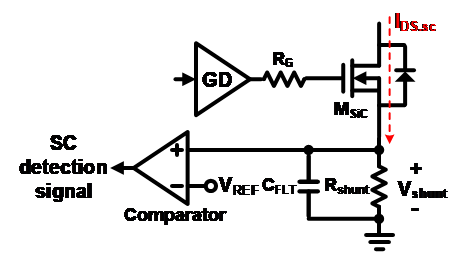##### Fig. 9. I$_{\mathrm{DS}}$ detection circuit using SenseFET.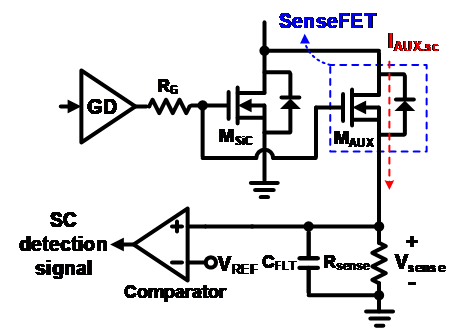##### Fig. 10. I$_{\mathrm{DS}}$ detection circuit by sensing voltage induced at parasitic inductance.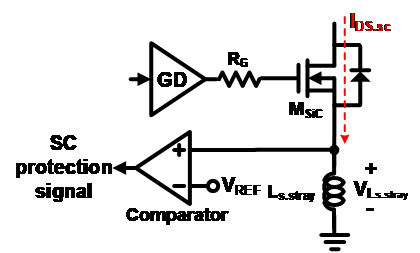##### Table 1. Comparison of Short Circuit Detection Circuits
 Short circuit detection circuit Method Gate-source voltage detection Drain-source voltage detection Drain-source current detection Implementation difficulty Medium Easy Hard Advantages No influence on the main current path High reliability and simple implementation Direct sensing of IDS Disadvantages Required filter to reduce noise Required external component Required parasitic stray inductance Accuracy Low accuracy due to Ciss of SiC MOSFET and RG variation  Low accuracy due to RG variation  High accuracy due to sensing of dIDS/dt Detection time under HSF state 300 ns  120 ns , 180 ns , 400 ns  60 ns , 150 ns  Detection time under FUL state - 20 ns  60 ns 

#### D. Hybrid Detection Method

Hybrid detection method combining the aforementioned circuits have also been proposed to improve the reliability of detecting the SC state of SiC MOSFETs operating at high speeds without error signals [32,33]. Fig. 11 illustrates a hybrid detection method that combines the V$_{\mathrm{GS}}$ detection method with the I$_{\mathrm{DS}}$ detection method . To prevent unwanted errors, the detection signal of the SC is generated to turn off the SiC MOSFET when the outputs of both detection circuits are logic ‘high’. Various combinations of circuits can be designed to ensure the reliability of the SC detection signal from the noise generated in the power system.

##### Fig. 11. SCDC combining the I$_{\mathrm{DS}}$ detection method and V$_{\mathrm{GS}}$ detection method for stability.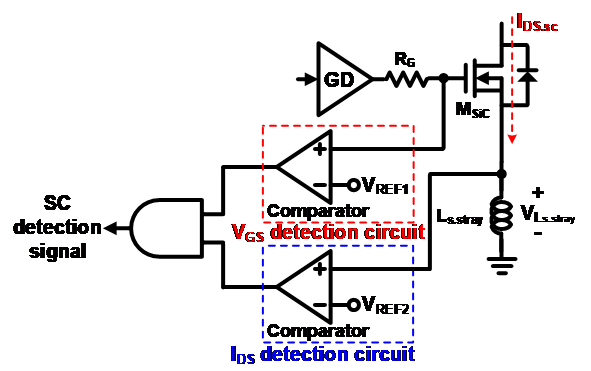## III. SOFT TERMINATION CIRCUITS

An STC is required to safely turn off the SiC MOSFET without damaging the power system due to the effects of the SC. Fig. 12 displays a diagram of the V$_{\mathrm{DS}}$ overshoot caused by a sudden drop in the V$_{\mathrm{GS}}$ in an SC situation. I$_{\mathrm{DS.SC}}$ decreases significantly if the SiC MOSFET (M$_{\mathrm{SiC}}$) is turned off without a separate safety technique when an SC current (I$_{\mathrm{DS.SC}}$) of several hundred Amperes flows. This sharp change of I$_{\mathrm{DS.SC}}$ induces a V$_{\mathrm{DS}}$ overshoot of L$_{\mathrm{d.stray}}$ ${\times}$ dI$_{\mathrm{DS.SC}}$/dt in the parasitic inductance L$_{\mathrm{d.stray}}$ by the PCB.

The overshoot voltage of V$_{\mathrm{DS}}$ can exceed the breakdown voltage of the SiC MOSFET device and destroy it. Moreover, the destruction of the SiC device may cause malfunctions in the power system. Therefore, an STC is required to turn off the device with a low dI$_{\mathrm{DS.SC}}$/dt when an SC state occurs. The most commonly used soft slope turn-off circuit and two-level turn-off circuit are as follows.

##### Fig. 12. Mechanism of V$_{\mathrm{DS}}$ overshoot when the SiC MOSFET turns off without STC in SC state.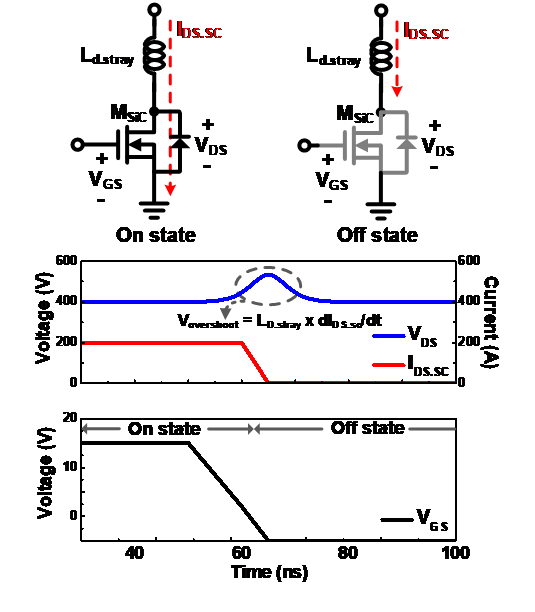### 1. Soft Slope Turn-off

The soft-slope turn-off circuit is depicted in Fig. 13 [21-23]. When an SC state is detected, the controller outputs a termination signal (V$_{\mathrm{detect}}$). At the time, the main driver operates in the off state and the auxiliary driver (M$_{\mathrm{sin}}$) reduces V$_{\mathrm{GS}}$. R$_{\mathrm{sink}}$ represents the resistance that determines the soft sinking current I$_{\mathrm{soft}}$; the value of R$_{\mathrm{sink}}$ ranges from several hundred ohms to several kilo ohms. The waveforms of the operation of the soft-slope turn-off circuit are depicted in Fig. 14. Once the V$_{\mathrm{detect}}$ signal reaches logic ‘high’, V$_{\mathrm{GS}}$ decreases during t$_{\mathrm{soft}}$, and the gain of the SiC MOSFET is lowered to suppress abrupt changes in dI$_{\mathrm{DS.SC}}$/dt. As depicted in Fig. 12, without the soft slope turn-off circuit, I$_{\mathrm{DS.SC}}$ has a fall time of several tens of nanoseconds; however, as observed in Fig. 14, since it is several hundred nanoseconds, the spike of V$_{\mathrm{DS}}$ can be arithmetically reduced by several orders of magnitude.

This circuit can effectively turn off an SiC MOSFET with a simple component. However, because it typically uses a fixed I$_{\mathrm{soft}}$, it is difficult to optimize the soft turn-off time for various SiC MOSFETs with different input capacitances proportional to their size and current capacity. Therefore, an additional circuit optimizing the soft turn-off times of various SiC MOSFET devices should be employed in a gate driver, which can drive the SiC MOSFET devices with suitable current capacities for each power application.

##### Fig. 13. Topology of a soft slope turn-off circuit.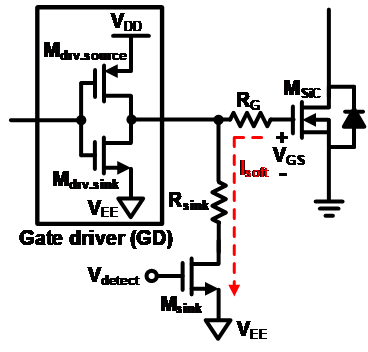##### Fig. 14. Waveforms of V$_{\mathrm{GS}}$, V$_{\mathrm{DS}}$, I$_{\mathrm{DS.SC}}$, and V$_{\mathrm{detect}}$ of a soft slope turn-off circuit.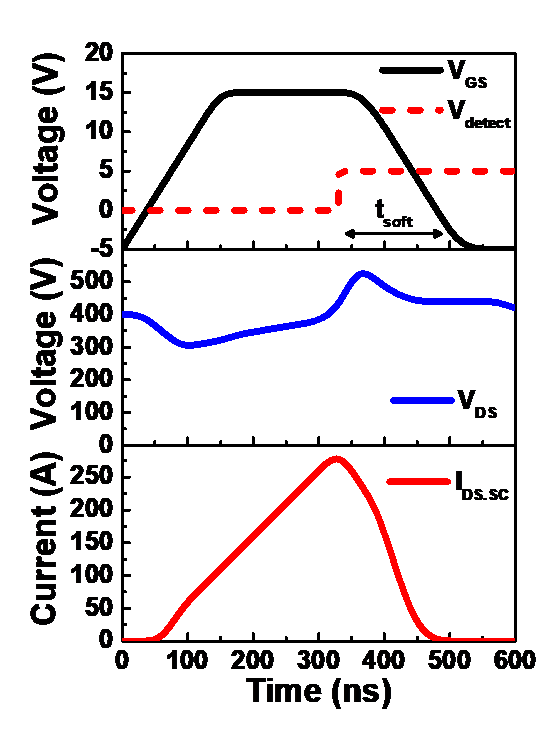### 2. Two-level Turn-off

The second circuit that is widely used as an STC includes the two-level turn-off circuit [20, 24, 34]. This circuit consists of a Zener diode (D$_{\mathrm{zener}}$) and sinking MOSFET (M$_{\mathrm{sink}}$), as shown in Fig. 15. Fig. 16 illustrates the soft turn-off operation shown in Fig. 15. When faults occur, M$_{\mathrm{sink}}$ is turned on, V$_{\mathrm{GS}}$ is clamped by the Zener voltage V$_{\mathrm{Z}}$ of D$_{\mathrm{zener}}$ and I$_{\mathrm{DS.SC}}$ decreases with a gentle slope. During t$_{\mathrm{soft}}$, the gain of the SiC MOSFET is suppressed so that I$_{\mathrm{DS.SC}}$ gradually decreases, and when it becomes sufficiently small, the main driver M$_{\mathrm{drv.sink}}$ operates to lower the V$_{\mathrm{GS}}$ by I$_{\mathrm{main}}$.

The two-level turn-off circuit is advantageous as it consistently has a constant soft turn-off time regardless of the current capacity of the SiC MOSFET, and it can configure the circuit using simple elements. However, as depicted in Fig. 16, overshoot voltages V$_{\mathrm{os1}}$ and V$_{\mathrm{os2}}$ occur in the two-stage clamping operation. Moreover, V$_{\mathrm{os1}}$ and V$_{\mathrm{os2}}$ may cause reliability problems in the SiC MOSFET depending on the design of PCB pattern. Table 2 summarizes and compares two previously mentioned methods of the soft termination circuit for SiC MOSFET.

##### Fig. 15. Topology of a two-level turn-off circuit.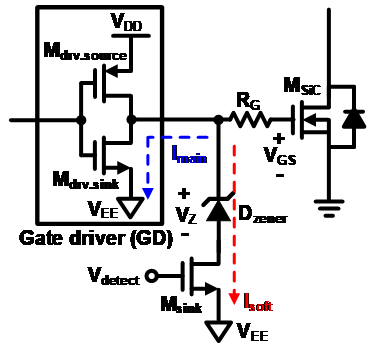##### Fig. 16. Waveforms of V$_{\mathrm{GS}}$, V$_{\mathrm{DS}}$, I$_{\mathrm{DS,SC}}$, and V$_{\mathrm{detect}}$ of a two-level turn-off circuit.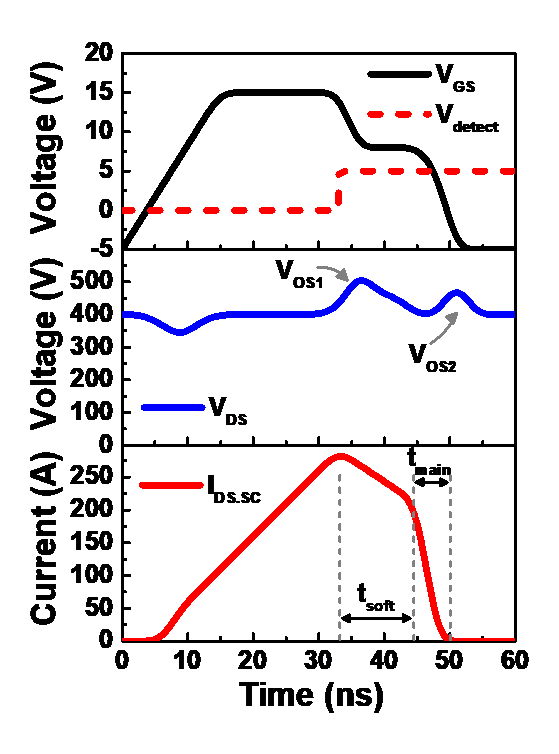##### Table 2. Comparison of Soft Termination Circuits
 Soft termination circuit Method Soft slope turn-off Two-level turn-off Advantages · Adjustable soft turn-off current · Easy on-chip implementation · Easy to reduce VDS spike · Soft turn-off time independent of Ciss of SiC MOSFET Disadvantages · Required customizing according to the capacitance value of Ciss of SiC MOSFET · Two VDS spike · Separate delay circuit required for soft turn-off time Soft turn-off time · Determined by Rsoft · Determined by tsoft

## IV. CONCLUSION

A short circuit protection circuit composed of a short circuit detection circuit and a soft termination circuit is required to protect the SiC MOSFET. This work reviewed the operation principles, advantages, and disadvantages of short circuit detection circuits and soft termination circuits. Moreover, the consideration for designing a short circuit protection circuit is introduced for a SiC MOSFET.

## ACKNOWLEDGMENTS

(MOTIE) This work was supported by the Korean Institute for Advancement of Technology (KIAT) grant funded by the Korean Government (P0012451, The Competency Development Program for Industry Specialist). This work was supported by the National Research Foundation of Korea (NRF) grant funded by the Ministry of Science and ICT (NRF-2019R1A2C1090935). The EDA tool was supported by the IC Design Education Center (IDEC), Korea.

## References

1
K. Hamada, M. Nagao, M. Ajioka, and F. Kawai, “SiC-Emerging power device technology for next-generation electrically powered environmentally friendly vehicles,” IEEE Transactions on Electron Devices, vol. 62, no. 2, pp. 278-285, Feb. 2015.2
J. He, A. Sangwongwanich, Y. Yang, K. Zhang, and F. Iannuzzo, “Design for reliability of SiC-MOSFET-based 1500-V PV inverters with variable gate resistance,” IEEE Transactions on Industry Applications, vol. 58, no. 5, pp. 6485-6495, Sept-Oct 2022.3
S. Hazra, A. De, L. Cheng, J. Palmour, M. Schupbach, B. A. Hull, S. Allen, and S. Bhattacharya, “High switching performance of 1700-V, 50-A SiC power MOSFET over Si IGBT/BiMOSFET for advanced power conversion applications,” IEEE Transaction on Power Electronics, vol. 31, no. 7, pp. 4742-4754, Jul. 2016.4
X. She, A. Q. Huang, Ó. Lucía, and B. Ozpineci, “Review of silicon carbide power devices and their applications,” IEEE Transactions on Industrial Electronics, vol. 64, no. 10, pp. 8193-8205, Oct. 2017.5
J. Rabkowski, D. Peftitsis, and H.-P. Nee, “Silicon carbide power transistors: A new era in power electronics is initiated,” IEEE Industrial Electronics Magazine, vol. 6, no. 2, pp. 17-26, 2012.6
J. Biela, M. Schweizer, S. Waffler, and J. W. Kolar, “SiC versus Si evaluation of potentials for performance improvement of inverter and DC-DC converter systems by SiC power semiconductors,” IEEE Transactions on Industrial Electronics, vol. 58, no. 7, pp. 2872-2882, 2011.7
“SiC MOSFETs : Gate drive optimization,” onsemi, Application Note TND6237/D, May. 2022.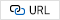8
J. Rice and J. Mookken, “SiC MOSFET gate drive design considerations,” in Proc. 2015 IEEE International Workshop on Integrated Power Packaging (IWIPP), May 20159
“CoolSiC 1200 V SiC MOSFET application note,” Infineon Technologies, Application Note AN2017-46, 2017.10
T. Horiguchi, S. Kinouchi, Y. Nakayama, and H. Akagi, “A fast short-circuit protection method using gate charge characteristics of SiC MOSFETs,” in Proc. 2015 IEEE Energy Conversion Congress and Exposition (ECCE), Sept. 2015.11
O. Climaco-Arvizu, L. Hernández-González, M. A. and Rodríguez-Blanco, “Fault detection for SiC-MOSFET based on the behavior of gate signal,” in Proc. 2015 IEEE 10th International Symposium on Diagnostics for Electrical Machines, Power Electronics and Drives (SDEMPED), Oct. 2015.12
Z. Wang, X. Shi, Y. Xue, L. M. Tolbert, F. Wang, and B. J. Blalock, “Design and performance evaluation of overcurrent protection schemes for silicon carbide (SiC) power MOSFETs,” IEEE Transactions on Industrial Electronics, vol. 61, no. 10, pp. 5570-5581, July. 2016.13
H. Li, Y. Wang, Z. Qiu, Z. Wang, X. Hu, J. Zhao, “Short-circuit protection circuit of SiC MOSFET based on drain-source voltage integral”, in Proc. IEEE Workshop on Wide Bandgap Power Devices and Applications in Asia (WiPDA Asia), Aug. 2021.14
D.-P. Sadik, J. Colmenares, G. Tolstoy, D. Peftitsis, M. Bakowski, J. Rabkowski, and H.-P. Nee, “Short-Circuit Protection Circuits for Silicon-Carbide Power Transistors,” IEEE Transactions on Industrial Electronics, vol. 63, no. 4, pp. 1995-2004, Apr. 2016.15
B. Zhou, D. Han, F. Z. Peng, S. Dwari, “Improved de-saturation protection circuits for silicon carbide MOSFET gate drivers,” in Proc. IEEE Applied Power Electronics Conference and Exposition (APEC), Jun. 2021.16
A. E. Awwad and S. Dieckerhoff, “Short-circuit evaluation and overcurrent protection for SiC power MOSFETs,” in Proc. Eur. Conf. Power Electron. Appl., Sept. 2015, pp. 1-9.17
M. Oinonen, M. Laitinen, and J. Kyyrä, “Current measurement and short-circuit protection of an IGBT based on module parasitics,” in Proc. Eur. Conf. Power Electron. Appl., Aug. 2014, pp. 1-9.18
J. Xue, Z. Xin, H. Wang, P. C. Loh, and F. Blaabjerg, “An improved di/dt-RCD detection for short-circuit protection of SiC MOSFET,” IEEE Transaction Power Electronics, vol. 36, no. 1, pp. 12-17, Jan. 2021.19
K. Sun, J. Wang, R. Burgos, and D. Boroyevich, “Design, analysis, and discussion of short circuit and overload gate-driver dual-protection scheme for 1.2-kV, 400-A SiC MOSFET modules,” IEEE Transactions on Power Electronics, vol. 35, no. 3, pp. 3054-3068, Mar. 2020.20
“Design Guide: TIDA-01605, Automotive Dual-Channel, SiC MOSFET Gate Driver Reference Design With Two-Level Turnoff Protection,” Texas Instruments, Application Note, Jun. 2019.21
M. Shim, K. Lee, J. Kim, and K. Kim, “Multistep soft turn-off time control to suppress the overvoltage of SiC MOSFETs in short-circuit state,” IEEE Access, vol. 10, pp. 46408-46417, Apr. 2022.22
J. Zhao, J. Zhang, H. Wu, Y. Zhang, “Design of gate driver and power device evaluation platform for SiC MOSFETS,” in 1st Workshop on Wide Bandgap Power Devices and Applications in Asia (WiPDA Asia), Jun. 2019.23
H. Zhou, C. Ye, X. Zhan, Z. Wang, “Designing a SiC MOSFETs gate driver with high dv/dt immunity and rapid short circuit protection for xEV drivetrain inverter,” in 22nd International Conference on Electrical Machines and Systems (ICEMS), Aug. 2019.24
A. Bhalla, S. Shekhawat, J. Gladish, J. Yedinak, and G. Dolny, “IGBT behavior during desat detection and short circuit fault protection,” in Proc. 10th International Symposium on Power Semiconductor Devices and ICs, Jun. 1998.25
R. S. Chokhawala, J. Catt, and L. Kiraly, “A discussion on IGBT short-circuit behavior and fault protection schemes,” IEEE Transactions on Industry Applications, vol. 31, no. 2, pp. 256-263, Mar.-Apr. 1995.26
S. Ji, M. Laitinen, X. Huang, J. Sun, W. Giewont, F. Wang, and L. M. Tolbert, “Short-circuit characterization and protection of 10-kV SiC MOSFET,” IEEE Transactions on Power Electronics, vol. 34, no. 2, pp. 1755-1764, Feb. 2019.27
“Understanding the Short Circuit Protection for Silicon Carbide MOSFETs”, Texas Instruments, Application note SLUA863, Jan. 2018.28
A. Niwa, T. Imazawa, R. Kojima, M. Yamamoto, T. Sasaya, T. Isobe, and H. Tadano, “A dead-time-controlled gate driver using current-sense FET integrated in SiC MOSFET,” IEEE Transactions on Power Electronics, vol. 33, no. 4, pp. 3258-3267, Apr. 2018.29
J. Person, M. Andresen, T. Rettmann, O. Mühlfeld, M. Liserre, “Short circuit detection methods for silicon carbide (SiC) power semiconductors,” in PCIM Europe 2019, Jul. 2019.30
S. Lee, K. Kim, M. Shim, I. Nam, “A digital signal processing based detection circuit for short-circuit protection of SiC MOSFET,” IEEE Transactions on Power Electronics, vol. 36, no. 12, pp. 13379-13382, Dec. 2021.31
R. Miftakhutdinov, X. Li, R. Mukhopadhyay, and G. Wang, “How to protect SiC FETs from short circuit faults - overview,” in Proc 20th European Conference on Power Electronics and Applications, Sept. 2018.32
P. Hofstetter and M.-M. Bakran, “The two-dimensional short-circuit detection protection for SiC MOSFETs in urban rail transit application,” IEEE Transactions Power Electronics, vol. 35, no. 6, pp. 5692-5701, Jun. 2020.33
W. Ouyang, P. Sun , M. Xie, Q. Luo, and X. Du, “A fast short-circuit protection method for SiC MOSFET based on indirect power dissipation level,” IEEE Transactions Power Electronics, vol. 37, no. 8, pp. 8825-8829, Aug. 2022.34
Z. Wang, X. Shi, Y. Xue, L. M. Tolbert, F. Wang, and B. J. Blalock, “Design and Performance Evaluation of Overcurrent Protection Schemes for Silicon Carbide (SiC) Power MOSFETs,” IEEE Transactions on Industrial Electronics, vol. 61, no. 10, pp. 5570-5581, Oct. 2014.Seungjik Lee received Lee received the B.S. degree in Electronic Engi-neering from Gyeongsang National University, Gyeongsang-nam-do, Korea, in 2019, and the M.S degrees in Electrical Engineering from Pusan National University, Busan, Korea, in 2021, and is currently working toward the Ph.D. degree in Electrical and Electronic Engineering at Pusan National University, Busan, Korea. His current research interests are CMOS RF/PMIC/analog circuits for wireless communications.

Ockgoo Lee received the B.S. degree in electrical engineering from Sungkyunkwan University, South Korea, in 2001, the M.S. degree in electrical engineering from the KAIST, South Korea, in 2005, and the Ph.D. degree in electrical and computer engineering from the Georgia Institute of Technology, USA, in 2009. Upon completion of the Ph.D. degree, he joined Qualcomm Inc., USA, as a Senior Engineer, where he was involved in the development of transmitters and integrated passive circuits on mobile applications. He is currently a Faculty Member of the Department of Electrical Engineering, Pusan National University, South Korea. His research interests include high-frequency integrated circuits and system design for wireless communications.

Ilku Nam received the B.S. degree in electronics engineering from Yonsei University, in Seoul, Korea, in 1999 and the MS. and Ph.D. degrees in Electrical Engineering and Computer Science from Korea Advanced Institute of Science and Technology (KAIST), in Daejeon, Korea, in 2001 and 2005, respectively. His Ph.D. work at KAIST was related to low-power CMOS RF transceiver integrated circuits (IC) design for low-power IoT applications. From 2005 to 2007, he was a Senior Engineer with RF development team, Samsung Electronics, Korea, where he was involved in developing world first multistandard multiband mobile digital TV tuner ICs supporting DVB-H/T, T-DMB, and ISDB-T/H. In 2007, he joined the Department of Electrical and Electronics Engineering, Pusan National University, Busan, Korea, where he is currently a Professor. From 2013 to 2014, he was an advisory professor with communication solution team, Samsung Electronics, Korea, where he was involved in the design of the 60 GHz WiGig circuits. His research interests include CMOS RF/mmWave/analog integrated circuits and RF security system for wireless communications such as IoT and 5G mobile systems.